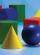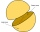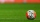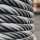# Solid geometry, stereometry

Solid geometry is the name for the geometry of three-dimensional Euclidean space.

Stereometry deals with the measurements of volumes of various solid figures (three-dimensional figures) including pyramids, prisms and other polyhedrons; cylinders; cones; truncated cones; and balls bounded by spheres.

1. Cone in cubeThe cube is inscribed cone. Determine the ratio of the volume of cone and cube. The ratio express as a decimal number and as percentage.
2. AquariumAquarium is rectangular box with square base containing 76 liters of water. Length of base edge is 42 cm. To what height the water level goes?
3. Cube wallSurface of first cube wall his 225 mm2. Second cube area is 60% of the surface of the first cube. Determine the length of the edge of the second cube (x).
4. Cube in ballCube is inscribed into sphere of radius 181 dm. How many percent is the volume of cube of the volume of sphere?
5. Hollow sphereCalculate the weight of a hollow tungsten sphere (density 19.3 g/cm3), if the inner diameter is 14 cm and wall thickness is 3 mm.
6. Plasticine ballPlasticine balls have radius r1=85 cm, r2=60 mm, r3=59 cm, r4=86 cm, r5=20 cm, r6=76 mm, r7=81 mm, r8=25 mm, r9=19 mm, r10=14 cm. For these balls
7. SandpileAuto sprinkled with sand to an approximately conical shape. Workers wanted to determine the volume (amount of sand) and therefore measure the circumference of the base and the length of both sides of the cone (over the top). What is the volume of the sa
8. Eiffel TowerEiffel Tower in Paris is 300 meters high, is made of steel. Its weight is 8000 tons. How tall is the tower model made ​​of the same material, if it weigh is 2.4 kg?The surface of the regular quadrilateral prism is 8800 cm2, the base edge is 20 cm long. Calculate the volume of the prism
10. Cutting coneA cone with a base radius of 10 cm and a height of 12 cm is given. At what height above the base should we divide it by a section parallel to the base so that the volumes of the two resulting bodies are the same? Express the result in cm.
11. Surface of pyramidIn a regular quadrilateral pyramid, the height of the sidewall is equal to the length of the edge of the base. The content of the sidewall is 32 cm2. What is the surface of the pyramid?
12. Height of pyramidThe pyramid ABCDV has edge lengths: AB = 4, AV = 7. What is its height?Calculate the surface of a quadrilateral pyramid, which has a rectangular base with dimensions a = 8 cm, b = 6 cm and height H = 10 cm.
14. Pentagonal prismThe regular pentagonal prism is 10 cm high. The radius of the circle of the described base is 8 cm. Calculate the volume and surface area of the prism.
15. Triangular prismCalculate the surface of a regular triangular prism, the edges of the base are 6 cm long and the height of the prism is 15 cm.
16. Two hemispheresIn a wooden hemisphere with a radius r = 1, a hemispherical depression with a radius r/2 was created so that the bases of both hemispheres lie in the same plane. What is the surface of the created body (including the surface of the depression)?
17. The hollow cylinderThe hollow cylinder has a height of 70 cm, an outer diameter of 180 cm and an inner diameter of 120 cm. What is the surface of the body, including the area inside the cavity?
18. What is bigger?Which ball has a larger volume: a football with a circumference of 66 cm or a volleyball with a diameter of 20 cm?
19. The conicalThe conical candle has a base diameter of 20 cm and a side of 30 cm. How much dm ^ 3 of wax was needed to make it?
20. Winch drumOriginally an empty winch drum with a diameter of 20 cm and a width of 30 cm on the rescue car, he started winding a rope with a thickness of 1 cm beautifully from edge to edge. The winch stopped after 80 turns. It remains to spin 3.54m of rope (without h

Do you have an interesting mathematical word problem that you can't solve it? Submit math problem, and we can try to solve it.

We will send a solution to your e-mail address. Solved examples are also published here. Please enter the e-mail correctly and check whether you don't have a full mailbox.

Please do not submit problems from current active competitions such as Mathematical Olympiad, correspondence seminars etc...